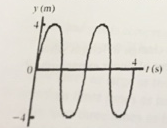# Problem: What is the wavelength of the wave shown in the figure? A) 8 m. B) 4 m. C) 2 m. D) 1 m. E) It cannot be determined from the given information.

###### FREE Expert Solution

The wave relationship:

$\overline{){\mathbf{v}}{\mathbf{=}}{\mathbf{f}}{\mathbf{\lambda }}}$

To determine the wavelength, we need to know v and f.###### Problem Details

What is the wavelength of the wave shown in the figure?A) 8 m.

B) 4 m.

C) 2 m.

D) 1 m.

E) It cannot be determined from the given information.